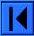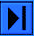The OEIS is supported by the many generous donors to the OEIS Foundation.Hints (Greetings from The On-Line Encyclopedia of Integer Sequences!)

## (Start)• This sequence of pages will show some of the ways that the On-Line Encyclopedia of Integer Sequences (or OEIS) can be used.
• Let's begin right away with an example of a beautiful sequence from the database, sequence A000037.
This entry illustrates most of the features that you will see in a typical OEIS entry.
(The following is a snapshot of the entry as it was on Sep 27 2011 — click the A-number to see the current version. The same thing applies to all the snapshots of sequences shown in these demonstration pages.)

 A000037 Numbers that are not squares. (Formerly M0613 N0223) 56
 2, 3, 5, 6, 7, 8, 10, 11, 12, 13, 14, 15, 17, 18, 19, 20, 21, 22, 23, 24, 26, 27, 28, 29, 30, 31, 32, 33, 34, 35, 37, 38, 39, 40, 41, 42, 43, 44, 45, 46, 47, 48, 50, 51, 52, 53, 54, 55, 56, 57, 58, 59, 60, 61, 62, 63, 65, 66, 67, 68, 69, 70, 71, 72, 73, 74, 75, 76, 77, 78, 79, 80, 82, 83, 84, 85, 86, 87, 88, 89, 90, 91, 92, 93, 94, 95, 96, 97, 98, 99 (list; graph; refs; listen; history; edit; internal format)
 OFFSET 1,1 COMMENTS Note the remarkable formula for the n-th term (see the FORMULA section)! These are the natural numbers with an even number of divisors. The number of divisors is odd for the complementary sequence, the squares (sequence A000290) and the numbers for which the number of divisors is divisible by 3 is sequence A059269. - Ola Veshta (olaveshta(AT)my-deja.com), Apr 04 2001 Also, a(n) = largest integer m not equal to n such that n = (floor(n^2/m) + m)/2. - Alexander R. Povolotsky (pevnev(AT)juno.com), Feb 10 2008 A010052(a(n)) = 0. [From Reinhard Zumkeller (reinhard.zumkeller(AT)gmail.com), Jan 26 2010] A173517(a(n)) = n; a(n)^2 = A030140(n). [From Reinhard Zumkeller (reinhard.zumkeller(AT)gmail.com), Feb 20 2010] Special case of formula from Farhi for positive integers which are not r-th powers [Jonathan Vos Post, May 5, 2011]. Union of A007969 and A007970; A007968(a(n)) > 0. [Reinhard Zumkeller, Jun 18 2011] REFERENCES A. J. dos Reis and D. M. Silberger, "Generating nonpowers by formula", Mathematics Magazine 63 (1990), pp. 53-55. J. Lambek and L. Moser, "Inverse and complementary sequences of natural numbers", The American Mathematical Monthly, Vol. 61, No. 7 (1954), 454-458, doi 10.2307/2308078, see example 4 (includes the formula). [From Nicolas Normand (Nicolas.Normand(AT)polytech.univ-nantes.fr), Nov 24 2009] M. A. Nyblom, "Some curious sequences involving floor and ceiling functions", American Mathematical Monthly 109 (2002), pp. 559-564. N. J. A. Sloane, A Handbook of Integer Sequences, Academic Press, 1973 (includes this sequence). N. J. A. Sloane and Simon Plouffe, The Encyclopedia of Integer Sequences, Academic Press, 1995 (includes this sequence). LINKS N. J. A. Sloane, Table of n, a(n) for n = 1..10000 Bakir Farhi, An explicit formula generating the non-Fibonacci numbers, May 5, 2011. S. R. Finch, Class number theory Eric Weisstein's World of Mathematics, Square Number Eric Weisstein's World of Mathematics, Continued Fraction FORMULA a(n) = n + [1/2 + sqrt(n)]. Another formula: a(n) = n + [ sqrt( n + [ sqrt n ] ) ]. a(n) = A000194(n) + n = floor(1/2 *(1 + sqrt(4*n-3)))+ n. [From Jaroslav Krizek (jaroslav.krizek(AT)atlas.cz), Jun 14 2009] d(a(n))=even. [From Juri-Stepan Gerasimov (2stepan(AT)rambler.ru), Oct 20 2009] a(n) = A000194(n) + n. EXAMPLE For example note that the squares 1, 4, 9, 16 are not included. a(A002061(n)) = a(n^2-n+1) = A002522(n) = n^2 + 1. A002061(n) = central polygonal numbers (n^2-n+1). A002522(n) = numbers of the form n^2 + 1. [From Jaroslav Krizek (jaroslav.krizek(AT)atlas.cz), Jun 21 2009] MAPLE A000037 := n->n+floor(1/2+sqrt(n)); MATHEMATICA f[n_] := (n + Floor[Sqrt[n + Floor[Sqrt[n]]]]); Table[ f[n], {n, 71}] (from Robert G. Wilson v Sep 24 2004) f[n_]:=Round[Sqrt[n]]; lst={}; Do[AppendTo[lst, n+f[n]], {n, 0, 5!}]; lst [From Vladimir Orlovsky (4vladimir(AT)gmail.com), Oct 13 2009] PROG (MAGMA) [n : n in [1..1000] | not IsSquare(n) ]; (MAGMA) at:=0; for n in [1..10000] do if not IsSquare(n) then at:=at+1; print at, n; end if; end for; (PARI) a(n)=if(n<0, 0, n+(1+sqrtint(4*n))\2) CROSSREFS Cf. A007412, A000005, A000290, A059269. Cf. A134986. Cf. A087153, A172151. [From Reinhard Zumkeller (reinhard.zumkeller(AT)gmail.com), Jan 26 2010] Sequence in context: A072099 A046841 A164514 * A028761 A028809 A028785 Adjacent sequences:  A000034 A000035 A000036 * A000038 A000039 A000040 KEYWORD easy,nonn,nice,changed AUTHOR N. J. A. Sloane (njas(AT)research.att.com), Simon Plouffe (simon.plouffe(AT)gmail.com) EXTENSIONS Edited by Charles R Greathouse IV (charles.greathouse(AT)case.edu), Oct 30 2009
Note the surprising formula for the n-th term!

•• To follow the sequence of pages, click only the direction buttonsat the bottom of the pages. (If you click on any of the active links you will have to use your browser's "Back" button to return here.)
• Although these demonstration pages are in English, note that the main lookup page has been translated into many other languages.
• The main URL for the database is http://oeis.org.
• The sequence of demonstration pages contains the following pages:

• Two other pages worth looking at are:Click the single right arrow to go to the next page.

Lookup | Welcome | Wiki | Register | Music | Plot 2 | Demos | Index | Browse | More | WebCam
Contribute new seq. or comment | Format | Style Sheet | Transforms | Superseeker | Recents
The OEIS Community | Maintained by The OEIS Foundation Inc.

Last modified May 24 19:38 EDT 2022. Contains 354043 sequences. (Running on oeis4.)